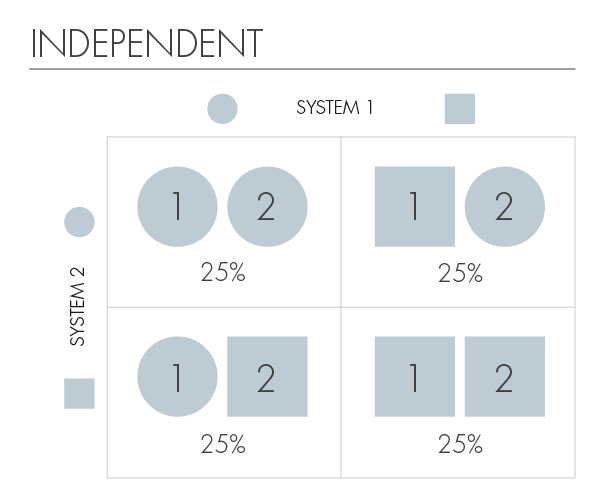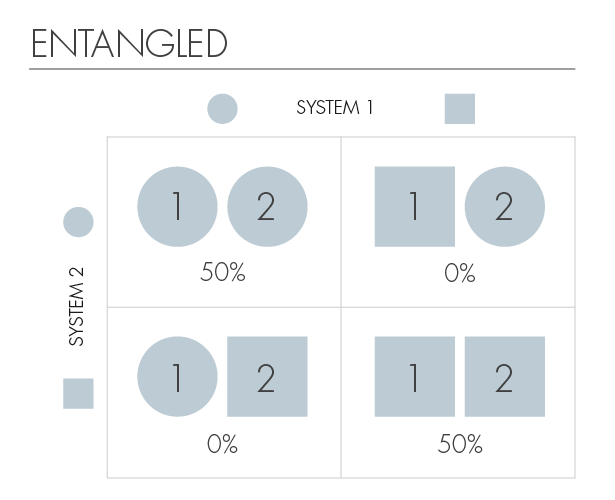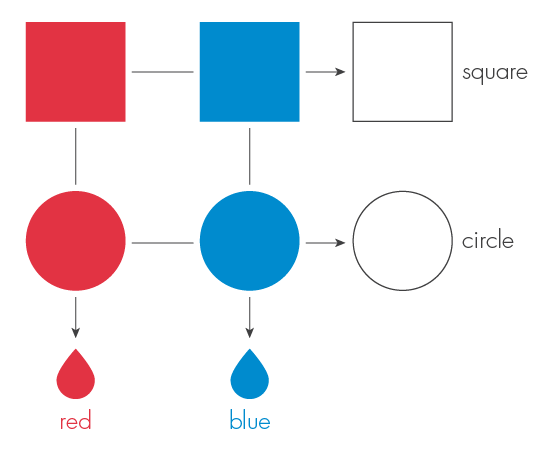I find the idea of entanglement useful, more for its analogies in how do teams work together. When people come together and then split apart… what portions of their interaction stay similar?

Pointer from: Reviewing notes from a weekend getaway
Place digested: Favorite seat at the dining Table
Time digested: Oct 23, 2018

By Frank Wilczek
April 28, 2016

# Entanglement Made SimplePART 1 & 2

I.
Entanglement is often regarded as a uniquely quantum-mechanical phenomenon, but it is not. In fact, it is enlightening, though somewhat unconventional, to consider a simple non-quantum (or "classical") version of entanglement first. This enables us to pry the subtlety of entanglement itself apart from the general oddity of quantum theory.

Entanglement arises in situations where we have partial knowledge of the state of two systems. For example, our systems can be two objects that we’ll call c-ons. The "c" is meant to suggest "classical," but if you’d prefer to have something specific and pleasant in mind, you can think of our c-ons as cakes.

Our c-ons come in two shapes, square or circular, which we identify as their possible states. Then the four possible joint states, for two c-ons, are (square, square), (square, circle), (circle, square), (circle, circle). The following tables show two examples of what the probabilities could be for finding the system in each of those four states.We say that the c-ons are "independent" if knowledge of the state of one of them does not give useful information about the state of the other. Our first table has this property. If the first c-on (or cake) is square, we’re still in the dark about the shape of the second. Similarly, the shape of the second does not reveal anything useful about the shape of the first.

On the other hand, we say our two c-ons are entangled when information about one improves our knowledge of the other. Our second table demonstrates extreme entanglement. In that case, whenever the first c-on is circular, we know the second is circular too. And when the first c-on is square, so is the second. Knowing the shape of one, we can infer the shape of the other with certainty.The quantum version of entanglement is essentially the same phenomenon — that is, lack of independence. In quantum theory, states are described by mathematical objects called wave functions. The rules connecting wave functions to physical probabilities introduce very interesting complications, as we will discuss, but the central concept of entangled knowledge, which we have seen already for classical probabilities, carries over.

Cakes don’t count as quantum systems, of course, but entanglement between quantum systems arises naturally — for example, in the aftermath of particle collisions. In practice, unentangled (independent) states are rare exceptions, for whenever systems interact, the interaction creates correlations between them.

Consider, for example, molecules. They are composites of subsystems, namely electrons and nuclei. A molecule’s lowest energy state, in which it is most usually found, is a highly entangled state of its electrons and nuclei, for the positions of those constituent particles are by no means independent. As the nuclei move, the electrons move with them.

Returning to our example:
If we write Φ, Φ for the wave functions describing system 1 in its square or circular states, and ψ, ψ for the wave functions describing system 2 in its square or circular states, then in our working example the overall states will be
Independent: Φ ψ + Φ ψ + Φψ + Φ ψ
Entangled: Φ ψ + Φ ψ
We can also write the independent version as
+ Φ)(ψ + ψ)

Note how in this formulation the parentheses clearly separate systems 1 and 2 into independent units.

There are many ways to create entangled states. One way is to make a measurement of your (composite) system that gives you partial information. We can learn, for example, that the two systems have conspired to have the same shape, without learning exactly what shape they have. This concept will become important later.

Previously, we imagined that our c-ons could exhibit two shapes (square and circle). Now we imagine that it can also exhibit two colors — red and blue. If we were speaking of classical systems, like cakes, this added property would imply that our c-ons could be in any of four possible states: a red square, a red circle, a blue square or a blue circle.

Yet for a quantum cake — a quake, perhaps, or (with more dignity) a q-on — the situation is profoundly different. The fact that a q-on can exhibit, in different situations, different shapes or different colors does not necessarily mean that it possesses both a shape and a color simultaneously. In fact, that "common sense" inference, which Einstein insisted should be part of any acceptable notion of physical reality, is inconsistent with experimental facts, as we’ll see shortly.

We can measure the shape of our q-on, but in doing so we lose all information about its color. Or we can measure the color of our q-on, but in doing so we lose all information about its shape. What we cannot do, according to quantum theory, is measure both its shape and its color simultaneously. No one view of physical reality captures all its aspects; one must take into account many different, mutually exclusive views, each offering valid but partial insight. This is the heart of complementarity, as Niels Bohr formulated it.

As a consequence, quantum theory forces us to be circumspect in assigning physical reality to individual properties. To avoid contradictions, we must admit that:
1. A property that is not measured need not exist.
2. Measurement is an active process that alters the system being measured.II.

Now I will describe two classic — though far from classical! — illustrations of quantum theory’s strangeness. Both have been checked in rigorous experiments. (In the actual experiments, people measure properties like the angular momentum of electrons rather than shapes or colors of cakes.)

Albert Einstein, Boris Podolsky and Nathan Rosen (EPR) described a startling effect that can arise when two quantum systems are entangled. The EPR effect marries a specific, experimentally realizable form of quantum entanglement with complementarity.
An EPR pair consists of two q-ons, each of which can be measured either for its shape or for its color (but not for both). We assume that we have access to many such pairs, all identical, and that we can choose which measurements to make of their components. If we measure the shape of one member of an EPR pair, we find it is equally likely to be square or circular. If we measure the color, we find it is equally likely to be red or blue.

The interesting effects, which EPR considered paradoxical, arise when we make measurements of both members of the pair. When we measure both members for color, or both members for shape, we find that the results always agree. Thus if we find that one is red, and later measure the color of the other, we will discover that it too is red, and so forth. On the other hand, if we measure the shape of one, and then the color of the other, there is no correlation. Thus if the first is square, the second is equally likely to be red or to be blue.

We will, according to quantum theory, get those results even if great distances separate the two systems, and the measurements are performed nearly simultaneously. The choice of measurement in one location appears to be affecting the state of the system in the other location. This "spooky action at a distance," as Einstein called it, might seem to require transmission of information — in this case, information about what measurement was performed — at a rate faster than the speed of light.
But does it? Until I know the result you obtained, I don’t know what to expect. I gain useful information when I learn the result you’ve measured, not at the moment you measure it. And any message revealing the result you measured must be transmitted in some concrete physical way, slower (presumably) than the speed of light.

Upon deeper reflection, the paradox dissolves further. Indeed, let us consider again the state of the second system, given that the first has been measured to be red. If we choose to measure the second q-on’s color, we will surely get red. But as we discussed earlier, when introducing complementarity, if we choose to measure a q-on’s shape, when it is in the "red" state, we will have equal probability to find a square or a circle. Thus, far from introducing a paradox, the EPR outcome is logically forced. It is, in essence, simply a repackaging of complementarity.

Nor is it paradoxical to find that distant events are correlated. After all, if I put each member of a pair of gloves in boxes, and mail them to opposite sides of the earth, I should not be surprised that by looking inside one box I can determine the handedness of the glove in the other. Similarly, in all known cases the correlations between an EPR pair must be imprinted when its members are close together, though of course they can survive subsequent separation, as though they had memories. Again, the peculiarity of EPR is not correlation as such, but its possible embodiment in complementary forms.